# R语言基于温度对城市层次聚类、kmean聚类、PCA主成分分析和Voronoi图可视化

## 最近我们被客户要求撰写关于聚类技术的研究报告。为了说明层次聚类技术和k-均值，我使用了了城市温度数据集，其中包括几个城市的月平均气温。

``````boxplot(temp[,1:12],main="月平均温度")
``````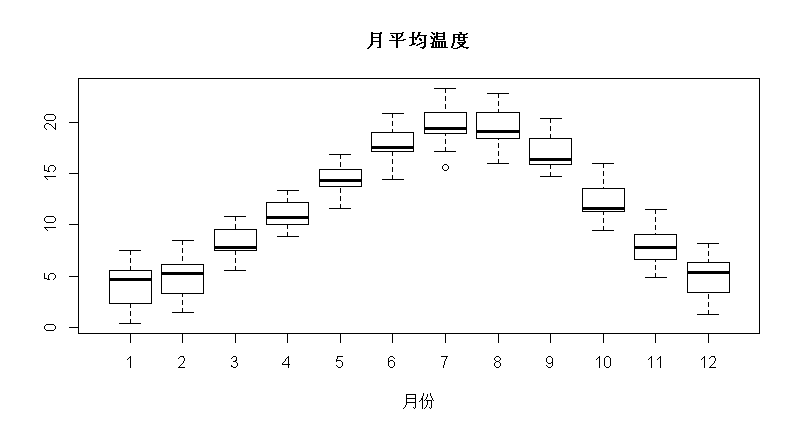``````> apply(月份,2,sd)
``````

KMEANS均值聚类和层次聚类：R语言分析生活幸福质量系数可视化实例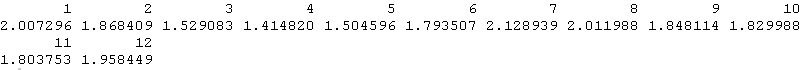`````` hclust(dist , method = "ward")
``````

``````
> plot(h2)``````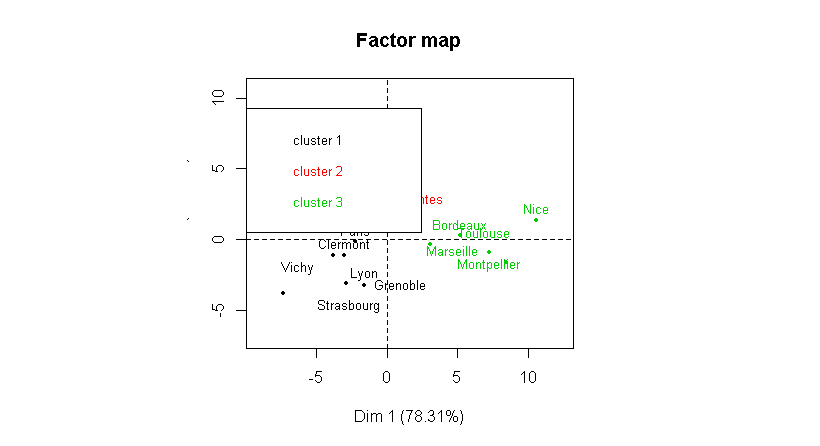`` cutree(cah,3)``

# r语言鸢尾花iris数据集的层次聚类

`````` PCA(X,scale.unit=FALSE)
plot(  ind\$coord[,1:2],col="white")
text( ind\$coord[,1],acp\$ind\$coord[,2],
``````

### 随时关注您喜欢的主题

`` > points(PT\$Dim.1,PT\$Dim.2,pch=19)``

``````vormo(PT\$Dim.1,PT\$Dim.2)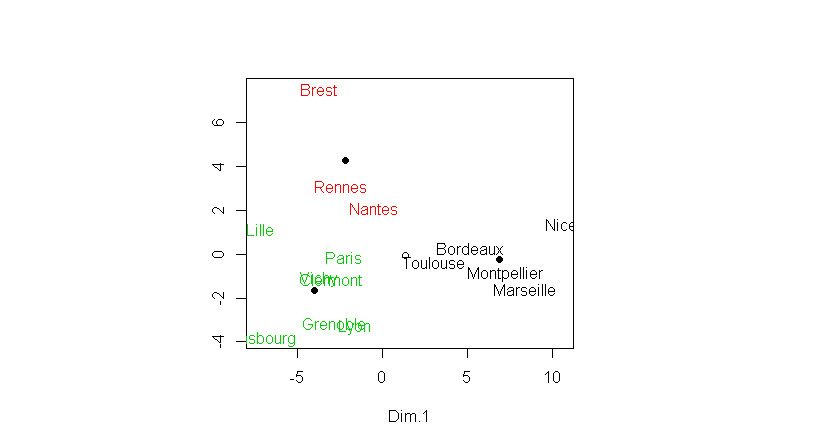# Voronoi图是由一组由连接两邻点直线的垂直平分线组成的连续多边形组成。

`````` p=function(x,y){
+   which.min((PT\$Dim.1-x)^2+(PT\$Dim.2-y)^2)
image(vx,vy,z,col=c(rgb(1,0,0,.2),
``````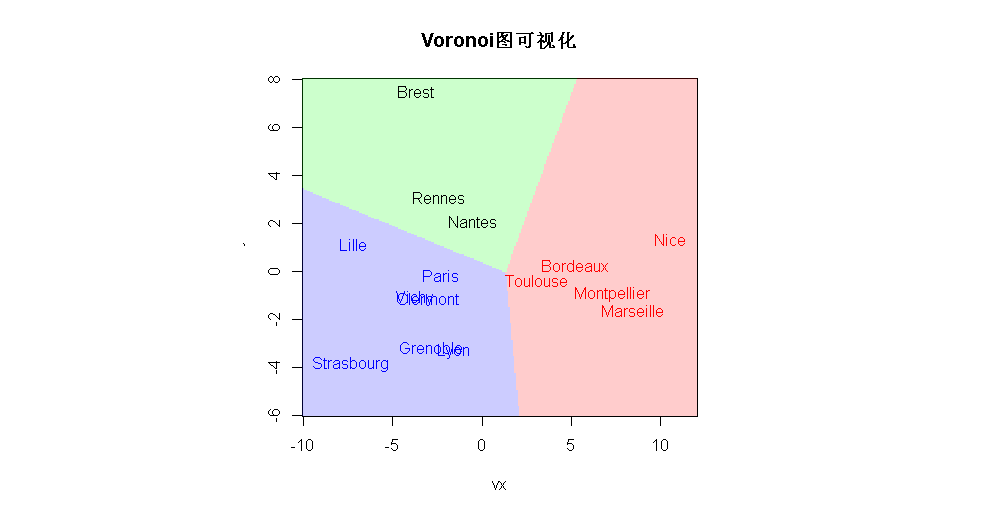# 实际上，这三组（和这三个区域）也是我们用k-均值算法得到的，

``````kmeans(coord[,1:2],3)

K-means clustering
with 3 clusters of sizes 3, 7, 5``````

``points(Long,Lati,col=groups.3)``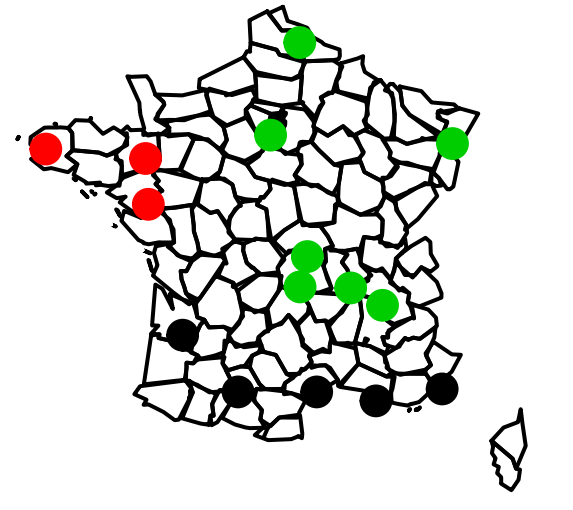`````` for(i in 1:3)
+ Ellipse( Long[groups.3==i],
``````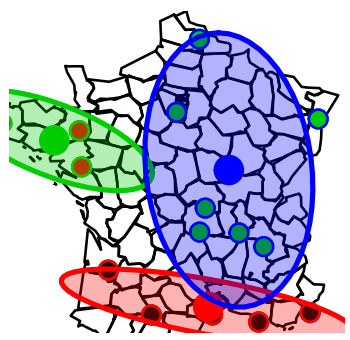Kaizong Ye拓端研究室（TRL）的研究员。

​非常感谢您阅读本文，如需帮助请联系我们！QQ在线咨询

15121130882

0571-63341498

## 关注有关新文章的微信公众号

This will close in 0 seconds